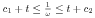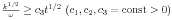WIAS Preprint No. 2078, (2015)

# Global-in-time existence of weak solutions to Kolmogorov's two-equation model of turbulence

Authors

• Mielke, Alexander
ORCID: 0000-0002-4583-3888
• Naumann, Joachim

2010 Mathematics Subject Classification

• 35K45 35Q30 76D03 76F99

Keywords

• Navier-Stokes equation, Kolmogorov's turbulence model, turbulent kinetic energy, global existence for weak solutions, defect measure, scaling laws, maximum principle

DOI

10.20347/WIAS.PREPRINT.2078

Abstract

We consider Kolmogorov's model for the turbulent motion of an incompressible fluid in ℝ3. This model consists in a Navier-Stokes type system for the mean flow u and two further partial differential equations: an equation for the frequency ω and for the kinetic energy k each. We investigate this system of partial differential equations in a cylinder Ω x ]0,T[ (Ω ⊂ ℝ3 cube, 0 < T < +∞) under spatial periodic boundary conditions on ∂Ω x ]0,T[ and initial conditions in Ω x {0}. We present an existence result for a weak solution {u, ω, k} to the problem under consideration, with ω, k obeying the inequalitiesand.

Appeared in

• C. R. Math. Acad. Sci. Paris, 353 (2015) pp. 321--326.